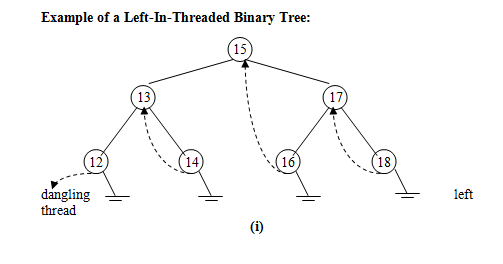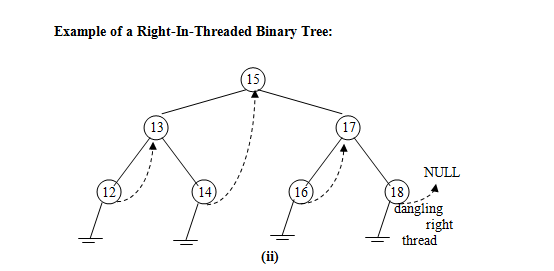Function for Inorder Traversal of an In-Threaded Binary Tree:

The basic advantage of Threaded Tree is that its inorder traversal does not require the use of stack.  Inorder traversal requires visiting the leftmost node, and then using right threads for the purpose of traversal.  The ‘C’ function for inorder traversal is given below:

/*Traverse in Inorder*/
void traverseInorder(tbt *root)
{tbt *temp=root;
while(temp->left)
temp=temp->left;
while(temp!=NULL)
{printf(“%dt”,temp->info);
if(temp->rflag==0)
{temp=temp->right;
while(temp->lflag==0)
temp=temp->left;
}
else
{temp=temp->right;
}
}
}

Function to insert a new node in In-Threaded Tree:

Insertion of a new node in a Threaded tree is quite similar to that of insertion in a Binary Search Tree with the only difference that at each insertion step, the inorder predecessor and inorder successor of the new node must be set accordingly and the root of newnode must be set to bear a subtree rather than a thread.  The ‘C’ function to insert a new node in a Threaded Binary Tree is given below:

/*Insert a newnode in the threaded tree*/
tbt *insert(tbt *root,tbt *newnode)
{
tbt *p,*temp=root;
if(root==NULL)
{root=newnode;
return root;
}
while(temp!=NULL)
{
if(newnode->info<temp->info)
{
if(temp->left)
{if(temp->lflag==1)
{
newnode->left=temp->left;
newnode->right=temp;
temp->left=newnode;
temp->lflag=0;
break;
}
else
temp=temp->left;
}
else
{newnode->left=temp->left;
temp->left=newnode;
temp->lflag=0;
newnode->right=temp;
break;
}
}
else
{if(temp->right)
{if(temp->rflag==1)
{newnode->right=temp->right;
newnode->left=temp;
temp->right=newnode;
temp->rflag=0;
newnode->rflag=1;
break;
}
else
{temp=temp->right;
}
}
else
{
p=temp->right;
temp->right=newnode;
temp->rflag=0;
newnode->right=p;
newnode->left=temp;
break;
}
}
}
return root;
}

Function to find the Inorder Predecessor of a given node in an In-Threaded Binary Tree:

Searching and returning inorder predecessor requires comparing each node with the value whose predecessor is required.  If the value is not found in the tree, error is reported.  If the value is found, then a temp pointer is placed on the left of this node and shifted until it points the rightmost node.  The value of the rightmost node is returned.  The ‘C’ function for returning Inorder predecessor of a given node is as follows:

/*Function that returns inorder predecessor*/
int inorderPredecessor(tbt *root,tbt *node)
{
int num;
tbt *p,*temp=root;
while(temp!=NULL)
{
if(node->info<temp->info)
{
if(temp->lflag==0)
temp=temp->left;
else /*move from left to right*/
temp=temp->right;
}
else if(node->info>temp->info)
{if(temp->rflag==0)
temp=temp->right;
else
{printf(“nGiven node does not exist.”);
return -1;
}
}
else/*if value is found*/
{if(temp->lflag==0)
{p=temp->left;
while(p->rflag!=1)
p=p->right;
num=p->info;/*predecssor*/
break;
}
else
{if(temp->left==NULL)
{num=0;
break;
}
else
{num=temp->left->info;
break;
}
}
}
}
return num;
}

Function to find the Inorder Successor of a given node in an In-Threaded Binary Tree:

Searching and returning inorder successor requires comparing each node with the value whose successor is required.  If the value is not found in the tree, error is reported.  If the value is found, then a temp pointer is placed on the right of this node and shifted until it points the leftmost node.  The value of the leftmost node is returned.  The ‘C’ function for returning Inorder Successor of a given node is as follows:

/*Function that returns Inorder successor*/
int inorderSuccessor(tbt *root,tbt *node)
{int num;
tbt *p,*temp=root;
while(temp!=NULL)
{
if(node->info<temp->info)
{if(temp->lflag==0)
temp=temp->left;
else
temp=temp->right;
}
else if(node->info>temp->info)
{
if(temp->rflag==0)
temp=temp->right;
else
{printf(“nGiven node does not exist.”);
return -1;
}
}
else/*node->info==temp->info*/
{
if(temp->rflag==0)
{
p=temp->right;
while(p->lflag!=1)
p=p->left;
num=p->info;/*successor*/
break;
}
else
{
if(temp->right==NULL)
{num=0;
break;
}
else
{num=temp->right->info;
break;
}
}
}
}
return num;
}

Function to traverse a Left-In-Threaded Tree in Descending Order:

Traversal of a Left In Threaded Tree result in a descending order of elements.  If the inorder traversal gives the result in Ascending order, then traversal using left threads may be performed such that the result is achieved in descending order.  The ‘C’ function for the same is as follows:

void traverseDescending(TBT *root)
{
TBT *p,*temp=root;
p=root;
while(temp!=NULL)
{while(p!=NULL)
{temp=p;
p=p->right;
}
printf(“%dt”,temp->info);
if((temp->lflag==1) && (temp->left==NULL))
return;
if(temp->lflag==1)
temp=temp->left;
else
p=temp->left;
}
}